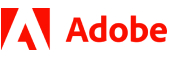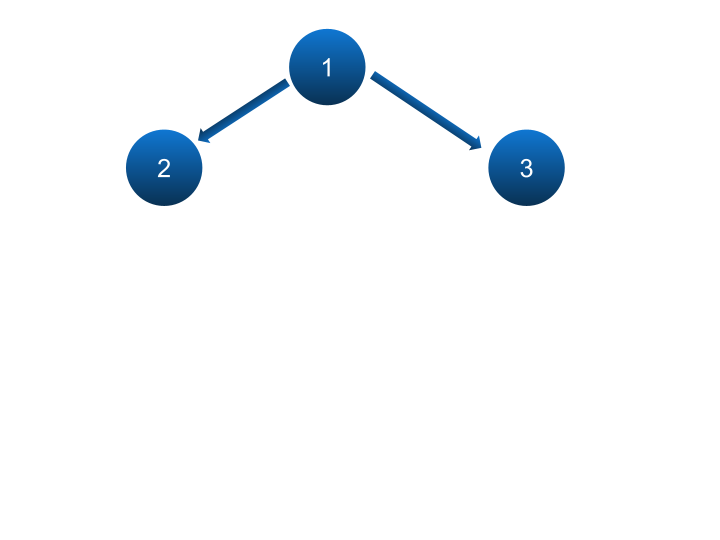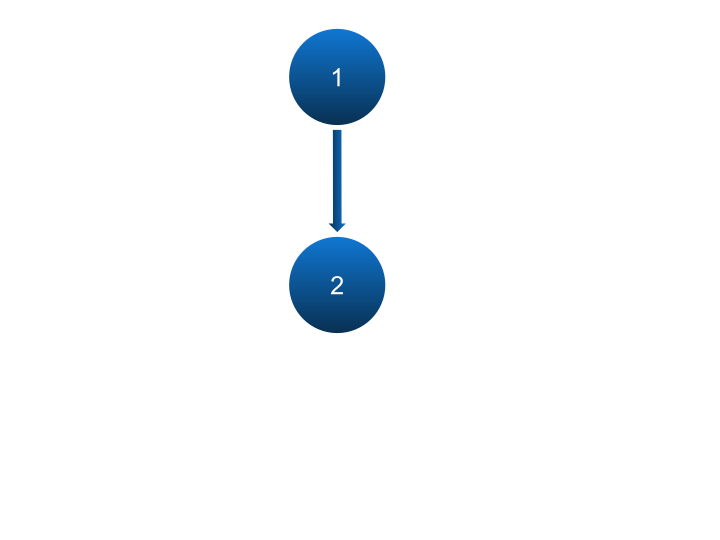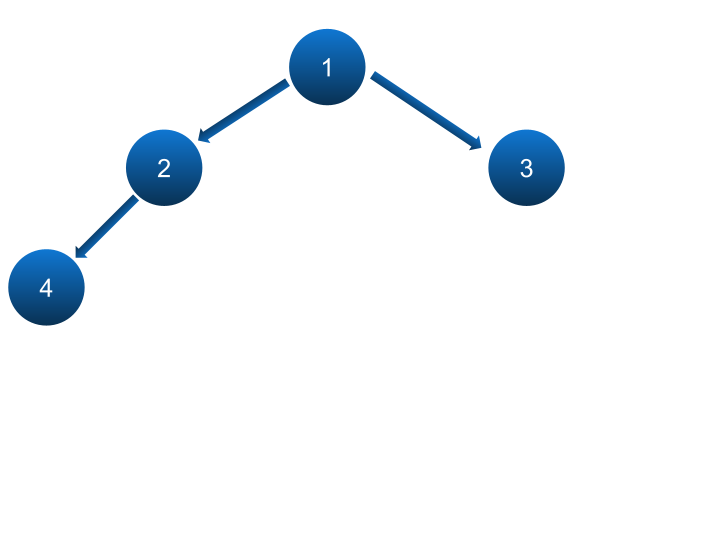New update is available. Click here to update.

# Ninja And Cities

Hard0/120
50 mins
50 %4 upvotes## Problem Statement

#### Now, you need to create an array of ‘N' - 1 elements where the ‘ith’ element is the number of subtrees in which the maximum distance between any two cities is equal to ‘i’.

##### Note:
``````1. Note that there exists a unique path between each pair of cites. Also, the final array that you need to create should be 1-indexed, meaning cities with a maximum distance = 1 should be stored on the normal 0th index.

2. Also, note that the distance between two is the number of cities you cross in between.
``````
##### Example:
``````Given 'N' = 7, and Array = {[1, 2], [1, 3], [2, 4], [2, 5], [3, 6], [3, 7]}

So the tree of cities formed in this case would look like:

In the above problem, the subtrees with subsets {[1, 2], [1, 3], [2, 4], [2, 5], [3, 6], [3, 7]}, have a maximum distance of 1, so put 6 on index 1.

Similarly the subtrees with subsets {[1, 2, 4], [1, 2, 5], [2, 1, 3], [4, 2, 5], [1, 3, 6], [1, 3, 7], [6, 3, 7], [1, 2, 4, 5], [1, 3, 6, 7]} have a maximum distance of 2, so put 9 on index 2.

Now, the subtrees with subsets {[4, 2, 1, 3], [2, 1, 3, 7], [5, 2, 1, 3], [2, 1, 3, 6], [4, 5, 2, 1, 3], [2, 1, 3, 6, 7]} have a maximum distance of 3, so put 6 on index 3.

Now, the subtrees with subsets {[4, 2, 1, 3, 7], [4, 2, 1, 3, 6], [5, 2, 1, 3, 7], [5, 2, 1, 3, 6], [5, 2, 1, 3, 6, 7], [4, 2, 1, 3, 6, 7], [4, 2, 5, 1, 3, 6], [4, 2, 5, 1, 3, 7], [4, 2, 5, 1, 3, 6, 7]} have a maximum distance of 4, so put 12 on index 4.

No subtree has two nodes where the maximum distance between them is 5 and 6 so print 0 on both these indexes.

Final resultant array = [6, 9, 6, 9, 0, 0]
``````
Detailed explanation ( Input/output format, Notes, Images )
##### Constraints:
``````1 <= T <= 10
2 <= N <= 15
size of array == N - 1
1 <= I1, I2 <= N

Time limit: 1 sec
``````
##### Sample Input 1:
``````1
3
1 2
1 3
``````
##### Sample Output 1:
``````2 1
``````
##### Explanation of Sample Output 1:
``````In test case 1, The tree of cities formed in this case would look like:
````````````In the above problem, the subtrees with subsets {[1, 2], [1, 3]} have a maximum distance of 1, so print 2.

Similarly, the subtrees with subsets {[2, 1, 3]} have a maximum distance of 2, so print 1.
``````
##### Sample Input 2:
``````2
2
1 2
4
1 2
1 3
2 4
``````
##### Sample Output 2:
``````1
3 2 1
``````
##### Explanation of Sample Output 2:
``````In test case 1, The tree of cities formed in this case would look like:
````````````In the above problem, the subtrees with subsets {[1, 2]} have a maximum distance of 1, so print 1.

In test case 2, The tree of cities formed in this case would look like:
````````````In the above problem, the subtrees with subsets {[1, 2], [1, 3], [2, 4]} have a maximum distance of 1, so print 3.

Similarly the subtrees with subsets {[1, 2, 4], [2, 1, 3]} have a maximum distance of 2, so print 2.

Now, the subtrees with subsets {[4, 2, 1, 3]} have a maximum distance of 3, so print 1.
``````AutocompleteConsole